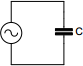Capacitive reactance calculator

Calculates AC properties of capacitance

 Example 1: Must calculate the reactance of a 2.2uF capacitor at 50Hz AC line View example Example 2: Must calculate the capacitance that have 10kohm reactance at 1kHz AC line View example
 Capacitance farads Frequency hertz Reactance ohmsYou may use one of the following SI prefix after a value: p=pico, n=nano, u=micro, m=milli, k=kilo, M=mega, G=giga

Fill in all values except the one you wish to calculate
• Capacitance - Capacitance of the component
• Frequency - Frequency of the circuit
• Reactance - Reactance of the circuit